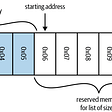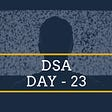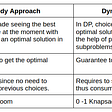Oh, bitmasks where do I start about those. One of the most difficult to understand topics in DSA (Data Structures and Algorithms) while simultaneously one that opens up entire new horizons for solving your problems. As someone who is constantly trying to improve my problem solving skills, bit masks were one of the easiest topics to learn, though it was the most unintuitive one as well. So I understand the mental block most people have towards this topic. In this blog I am going to walk you through some basic bit concepts and how to apply bit masks in a DSA question. And by the time you are done with this you will be able to comprehend at least a little bit of what those 1’s and 0’s that have been used in a recursive function mean. I will also solve a leetcode problem to guide you through this.

# Basics

So before I start with the problem, lets make sure that you have the basics down. If you already have an idea about how the bitwise operators work feel free to skip this part. I will be using python for this but it can be reproduced in any language.

## 1. Bitwise AND ( & ):

The “bitwise AND” operator performs the logical “AND” operation on the bits. This basically means that for 2 numbers if both the bits at the same position are 1, the output will be one, else 0. Let me explain this with a short example.

`num1 = 1 1 0 0 1 0 0 1num2 = 1 0 0 1 0 0 1 0ans  = 1 0 0 0 0 0 0 0`

This is all good but how is it used in a programming context? The bitwise and operator is used when you want to check whether a certain bit is 1 or not. You basically perform the AND operation between the bit you want to check and a number that has 1 at that specific bit and 0 at every other bit. If the bit is 1 the output will be a non zero number, else it will be 0. Take a look at the examples below for better understanding.

`num1 = 1 1 0 0 1 0 0 1num2 = 1 0 0 0 0 0 0 0ans  = 1 0 0 0 0 0 0 0num1 = 1 1 0 0 1 0 0 1num2 = 0 0 0 1 0 0 0 0ans  = 0 0 0 0 0 0 0 0`

As you can see in the first example since the desired bit was 1 the answer was non zero, while in the second example since the desired bit was 0 the answer was 0. Since all the bits except the one that needs to be checked are 0 in num2 it matters not what the other bits in the number were, since 0 & 1 = 0.

Another way the AND operator can be used is like an “off switch”. It is used to update a bit to 0, regardless of what the initial state of the bit was. This is done by performing the AND operation with a number with 0 in the bit that needs to be switched off and 1 at all the other positions. Take a look at the below example for better understanding.

`num1 = 1 1 0 0 1 0 0 1num2 = 1 0 1 1 1 1 1 1ans  = 1 0 0 0 1 0 0 1num1 = 1 1 0 0 1 0 0 1num2 = 1 1 1 0 1 1 1 1ans  = 1 1 0 0 1 0 0 1`

In the first example since the bit to be turned off is a 1 it is updated to 0, while in the second example the bit remains unchanged since it already is a 0. The other bits are unchanged because bit & 1 = bit. Though this use of the AND operator is seldom, as it is relatively difficult to generate a binary number with 0 at a particular bit and all other 1's than it is to generate one with 1 at a particular bit and all other 0's.

## 2. Bitwise OR ( | ):

The “bitwise OR” operator performs the logical “OR” operation on the bits. This basically means that for 2 numbers if any one of the bits at the same position is 1, the output will be one, else 0. Let me explain this with a short example.

`num1 = 1 1 0 0 1 0 0 1num2 = 1 0 0 1 0 0 1 0ans  = 1 1 0 1 1 0 1 1`

The bitwise OR operator is mainly used as an “on” switch. Similar to the and operator, it is used to update a bit to 1, regardless of what the prior state of the bit was. This is done by performing the OR operation between the bit you want to turn on and a number with 1 at that particular bit and 0 at all the other bits. Take a look at the examples below for better understanding.

`num1 = 1 1 0 0 1 0 0 1num2 = 1 0 0 0 0 0 0 0ans  = 1 1 0 0 1 0 0 1num1 = 1 1 0 0 1 0 0 1num2 = 0 0 0 1 0 0 0 0ans  = 1 1 0 1 1 0 0 1`

In the first example since the bit to be “switched on” is already a 1, it remains as a one, while in the second one the bit is a 0 so it is turned into a 1. And since all the other bits in num2 are 0, in the final answer they are not changed, since bit | 0 = bit.

## 3. Bitwise XOR ( ^ ):

We have already seen an “on switch” and an “off switch”, but what if we want a switch that can do both? That’s where the bitwise XOR operation comes in place. This basically toggles a bit between the “on” and “off” i.e. 1 and 0 state. So a 1 becomes 0 and a 0 becomes 1. The XOR operation basically gives 0 as the output if both the bits at the same position are the same ( both 0 or both 1), else it gives 1 as an output.

`num1 = 1 1 0 0 1 0 0 1num2 = 1 0 0 1 0 0 1 0ans  = 0 1 0 1 1 0 1 1`

This is done by performing an XOR operation with a number with 1 in the desired bit and all other bits as 0. Take a look at the below example for better understanding.

`num1 = 1 1 0 0 1 0 0 1num2 = 1 0 0 0 0 0 0 0ans  = 0 1 0 0 1 0 0 1num1 = 1 1 0 0 1 0 0 1num2 = 0 0 0 1 0 0 0 0ans  = 1 1 0 1 1 0 0 1`

In the first example, since the bit is 1 it is updated to 0, while in the second example it changes from 0 to 1, thus the bits are toggled. All the other bits are unchanged because bit ^ 0 = bit.

## 4. Left Shift ( << ) and Right Shift ( >> ):

Compared to the operations above, the left and right shift operations are fairly easy to understand. They just shift the bit to the left or right by certain amounts. You can think of a binary number being an infinitely long string of 0’s with 1’s at the required positions. The shift operators just shift the string to the right or left. Take a look at the below example.

Let the number be 00010. Then number << 2 will give 01000. The bits shifted to the left by 2 i.e. the number was multiplied by 4 ( 2² ), the power is the amount it is shifted.

Let the number be 01000. Then number>>2 will give 00010. The bits were shifted to the right by 2 i.e. the number was divided by 4 ( 2² ), the power is the amount it is shifted.

These right and left shift operators are mainly used to create binary strings with 1 at a specific bit to act as a helper number for the AND, OR and XOR operations.

# Problem:

I hope by now the basic bitwise concepts have been cleared. To show you a demonstration of how these operators work together, I will solve a Leetcode problem which uses these concepts.

The problem I am going to solve is the Maximum Compatibility Score sum. You can check it out over here.

This problem is basically about finding out the maximum score between the students and mentors, a classic DP problem which can be solved by using bitmasks, in place of the array that is used to keep track of the nodes (in this case mentors) visited/checked.

Take a look at the code below.

So the first part of the code is quite easy to understand we are just creating a compatibility score matrix between the students and mentors. The part which we are concerned about is the “getscore” function, and how the “seen” argument which is our bitmask is used in this case. In this case we are using the bitmask in place of an array of boolean values. The ones correspond to True while the zeroes correspond to False. As you can see in the example below the bitmask is essentially equivalent to the boolean array. That’s the way you form an equivalence between the two.

`1 1 0 1 0 0 1T T F T F F T`

So as you can see on line 17 of the code block above we perform an AND operation between the seen bitmask and 1<<j. This 1<<j just creates a binary number with 1 at the j’th bit. We use this to find out if the bit at that position in seen is 1 or 0. If it is 1 the output will be non-zero, and if it is 0 the output will be zero, in which case the if statement will be executed. We do this because we don’t want to consider the same mentor again and again.

Now on line 18 we perform an OR operation between seen and 1<<j. In this case this will switch on the bit at the j’th position and give us an updated bitmask. This means that it will not consider the mentor at the j’th position for further calculation.

That’s it for all the bitmask related content. I know that I didn’t explain the problem clearly but that was not the focal point of this blog. If you want to learn more about this problem check out this awesome youtube video by Larry.

This was just for bitmasks, but if you ever find yourself stuck while learning a DSA concept try to convert it into such a problem, you can even try browsing leetcode for it. It helps a lot in the understanding process.

Burhanuddin Rangwala the author of this blog is an aspiring Data Scientist and Machine Learning Engineer who also works as a freelance web developer and writer. Hit him up on linkedIn for any work or freelance opportunities.

--

--

--

## More from Burhanuddin Rangwala

Aspiring data scientist and ML enthusiast

Love podcasts or audiobooks? Learn on the go with our new app.

## Lists and Tuples in Python## Kubeflow Tips & Tricks — February 23rd, 2022## Locust experiments — Feeding the locusts## DevOps ZABBIX TooL## Maximising RoI from available technologies## Developer Update: 4.0.14## Ideal Cloud Hosting Plans at Low Prices## Burhanuddin Rangwala

Aspiring data scientist and ML enthusiast

## Greedy Algorithms## Comparison of Algorithms-KNN vs Naive Bayes## What is “Tree Data Structure”## Longest Increasing Subsequence